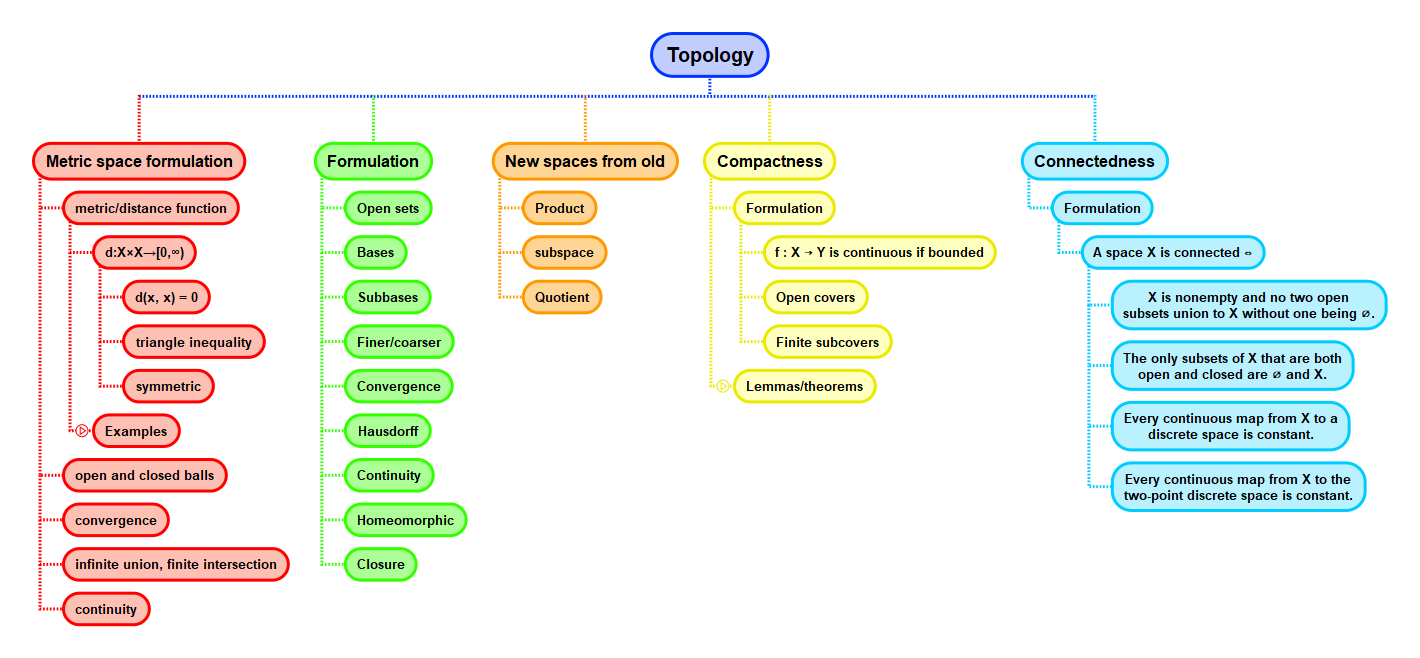Show Question
Math and science::Topology

# Neighbourhoods

Let $$X$$ be a set with topology $$\mathcal{T}$$. A neighbourhood of $$x$$ is an open subset of $$X$$ containing $$x$$.

This is the definition according to Munkres. Tom Leinster gives a different definition that distinguishes between a 'neighbourhood' and an 'open neighbourhood'.

Neighbourhood is the closest we get to the metric space idea of open balls around a point.

The term neighbourhood packs a noun-verb pair into a noun, which I think is part of why using it makes it easier to conceptualize compared to 'open set containing $$x$$'.

The next lemma is of interest because it is a lemma expressed in the terms of topology that is in some sense an isomorphism of a definition that appeared when formulating open sets in metric spaces. When defining open sets of a topology, for our definition we took the metric space's derived result of open sets being closed under arbitrary union and finite intersection. Now we have come to a derived result of topological spaces that matches the founding definition of open sets in metric spaces. Isn't that an interesting loop.

### Lemma. Open iff all elements have a neighbourhood subset

Let $$X$$ be a topological space and $$U \subseteq X$$. Then $$U$$ is open in $$X$$ iff for all $$x \in U$$, there is a neighbourhood of $$x$$ contained in $$U$$.

For comparison, the definition of open sets for a metric space is copied below:

#### Metric space: Open subsets, definition

Let $$X$$ be a metric space and $$U \subseteq X$$. Then $$U$$ is open in $$X$$ iff for all $$u \in U$$, there is a $$\varepsilon > 0$$ such that $$B(u, \varepsilon) \subseteq U$$.

## Context